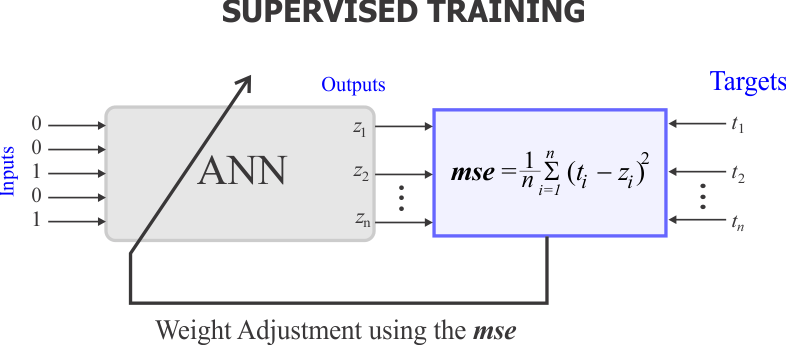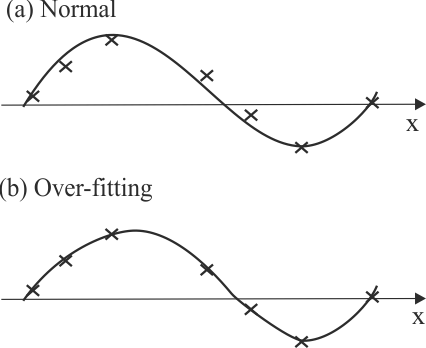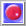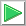# Validation

## Validation

After the ANN training has been completed. The network performance has to be validated. Typically, the validation process is performed using another data set called the validation set.

## Validation Set

The validation set is used after the neural network has been trained to assess its performance. The validation set is similar to the training set but not equal. Many practitioners of ANN forget to validate the trained neural network using the validation set.

 Tip A typical mistake that can be done when using artificial neural networks is to use the validation set for training. This is similar to a student getting a copy of a test before taking it. The network would learn from the data set and incorporate it into its strategy to achieve perfect accuracy, much like a student in a similar situation might do. However, with a different data set may not perform well at all, even if they are similar. More problematic is that there would be no way of telling whether the network is performing well.

 Tip The purpose of these two sets (the training set and the validation set) is to assess how well the neural network will behave with other sets in real life applications. As a general rule, the training set must include all possible training cases. This will guarantee that the neural network will behave similarly with the validation and the training set. In some real life problems, however, it is not possible to include all possible cases in the training set.

## Supervised Training

To monitor the training process, the output of an ANN can be compared with the desired output (target). This kind of training is called supervised training as the ANN adjusts its behavior to achieve the target value for each training case.

## MSE

To assess the quality of the behavior of an artificial neural network, the mean squared error (mse) is typically used for comparison purposes. It is computed between the actual network output and the target. The figure shown below illustrates how the mse is computed and used to adjust the network weights.Tip Many students have trouble deciding how many neurons and layers a neural network should have to solve a specific problem. Typically, the number of hidden neurons can be increased to reduce the mse. However, this must be done with caution as machine learning over-fitting may occur. It is very easy to commit over-fitting because many tools to simulate ANN do not caution the user when this happens. If the mse obtained during training is much smaller that the mse obtained during validation, over-fitting has been committed (see figure below); in this case the training and the neural network are useless.Tip Never train an ANN using less than the minimum of training cases.

 Problem 1 The ANN shown below has been simulated using two input training cases as shown. Compute manually the mse.Problem 2 Repeat the last problem using Neural Lab.

 Solution 2Neural Lab open Neural Lab, create a New Project called ComputeMse, and then write the code show below.Run click the button to execute the code. You will be asked to save the file, choose an appropriate folder and file name. If you do not have any errors, the mse will be computed and displayed.

 ComputeMse\Main.lab Matrix w; w.Create(2, 3); w = -4.8; w = 4.6; w = -2.6; // w = 5.1; w = 5.2; w = -3.2; LayerNet net; net.Create(2, 0, 0, 2); net.SetWeights(3, w); Matrix trainSetInput; trainSetInput.Create(2,2); //__________________ training case 0 trainSetInput = 0.1; trainSetInput = 0.5; //__________________ training case 1 trainSetInput = 0.2; trainSetInput = 1.1; Matrix output = net.Run(trainSetInput); Matrix trainSetTarget; trainSetTarget.Create(2,2); //__________________ training case 0 trainSetTarget = -0.9; trainSetTarget = -0.2; //__________________ training case 1 trainSetTarget = 0.9; trainSetTarget = 0.8; double mse = ComputeMse(output, trainSetTarget);

 Problem 3 A student trained an ANN using 128 training cases. When he used the training set, the mse was 0.001. When he used the validation set, the mse was 0.02. What can be concluded from the experiments?

 Problem 4 A student trained an ANN using 256 training cases; the ANN had 10 neurons in the hidden layer. When he used the training set, the mse was 0.01. When he used the validation set, the mse of the same network was 0.0001. What can be concluded from the experiment?

 Problem 5 A student trained an ANN using 1024 training cases. When using 10 neurons in the hidden layer, the mse using the training set was 0.0002. The mse of the same network but using the validation set was 0.0003. He reduced the number of neurons in the hidden layer to 5, and the mse for training a validation remained very similar to their previous values. What can be concluded from the experiment?

 Problem 6 A homework assignment included the optimum design of an ANN with 5 inputs, 6 outputs and one hidden layer. The professor suggested to begin the experiment with one neuron in the hidden layer, and compute both the mse for training and for validation. The students were told to increase the number of neurons in the hidden layer and compute again the mse for training and for validation. The students kept on increasing the number of neurons in the hidden layer. At what point should they stop the experiment?

 Problem 7 A student was building an ANN in hardware as shown below. A temperature problem damaged the memory location of one of the weights of the network. If the mse before failure was 0.465589, what was the value of the weight? Hint: You can compute the value of z1 for the first training case. Similarly, compute the value of z1 for the second training case. Then, compute the value of z2 for the first training case as a function of the missing weight. Compute the value of z2 for the second training case as a function of the missing weight. Finally, find the value of the missing weight using a numerical method (algorithm)Problem 8 Write some code to solve last problem. Create an ANN with 2 inputs, two outputs, and zero hidden layers. Set manually all the given weights. Generate randomly the missing weight until the mse is the specified value. Assume that the network weights can take values from -30.0 to 30.0. Your project must be called ComputingWeight.

 ComputingWeight.lab Matrix w; w.Create(2, 3); ... LayerNet net; net.Create(2, 0, 0, 2); Matrix itrainSetInput; ... Matrix trainSetTarget; trainSetTarget.Create(2, 2); .. double mse = 100.0; Matrix output; while (mse < 0.465588 || mse > 0.465590) {      ...      }

## Main Steps to use an ANN

1. Build the training set (input and target)
2. Build the validation set (input and target)
3. Design the network (Set the network input and output ranges)
4. Train the network (Set training methods and training parameters)
5. Check the training (compute the mse for the training set or classify the cases)
6. Perform the validation (compute the mse for the validation set or classify the cases)
7. Analyze and review the experiments and their results
8. Use the network

 Problem 9 During the training of an ANN the mse was 0.1. If the output value has a mean of 0.9, what would be the minimum and maximum values expected at the output of the network?

 Tip When the validation has been completed, you must use all cases to train the network before using it for production.